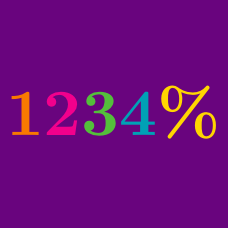Everyday Math

# Percentages Warmup

Which is larger:

$A = \frac{90\text{\%}}{90\text{\%}}$

$B = 90\text{\%} \times 90\text{\%}$

What is greater?

A: 200% of 50

B: 50% of 200

If X is positive, when does multiplication equal addition?

$X\text{\%}\times X\text{\%}= X\text{\%} + X\text{\%}$

$X = ?$

$\huge \frac{100\text{\%}}{10\text{\%}} \ = \ ?$

$\large \sqrt{X\text{\%}} = X\text{\%}$

Solve for X where X is positive.

×